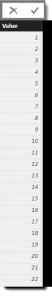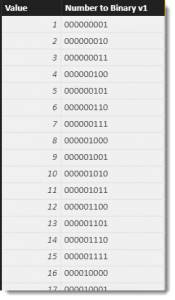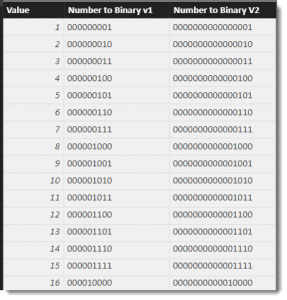# Quick DAX : Convert number to binary (and back)

Posted on Jun 27, 2018 in DAX, Power BI

Something that is currently missing in DAX is a native set of functions to perform Bitwise operations.  These can be incredibly useful functions and one, in particular, is the ability to convert a  base10 number to its binary format. Once you have the number in binary format, you can apply logical operations between numbers to determine the various state of each bit that can represent a flag.

To demonstrate, I generate a simple table of numbers using the GENERATESERIES function.  This table will have a sequence of numbers between 1 and 512 that will be used to convert to binary.

This creates the following calculated table that I will use to demonstrate my “Number to Binary” calculation by adding some columns that show the binary representation of the number in the first column.First up, here is the extended version of the calculated column used to convert numbers into their binary equivalent.  Note the number of “Bits” calculated is controlled by the number of lines in the RETURN statement.

The function works by combining the DIVIDE and MOD functions to return a 1 or 0 depending on the position of the bit.  The order is controlled by the order in the concatenation.If more bits need to be calculated, then more lines can be added to the return statement OR the CONCATENATEX function can be used to parameterise the approach

This version allows you to set a value for the Bits variable to control how many bits should be calculated.While the output of both the V1 and V2 calculations are text, this now makes it possible to create Bitwise operations over the text output using the SUBSTRING function to extract the individual bits to then simulate AND, OR, and XOR type operations.

To convert a binary value back to dec (base 10), just find the row that matches your binary value and return the number from the first column.

The PBIX File used for this blog can be downloaded here :

https://1drv.ms/u/s!AtDlC2rep7a-pmXEJQwoBkdzuzuK

Phil is Microsoft Data Platform MVP and an experienced database and business intelligence (BI) professional with a deep knowledge of the Microsoft B.I. stack along with extensive knowledge of data warehouse (DW) methodologies and enterprise data modelling. He has 25+ years experience in this field and an active member of Power BI community.

•david lyth says:

2 to the power of 0 = 1, so you can tidy up the V2 slightly:
N2B V3 =
VAR Number = ‘Binary Mapping'[Value]
VAR Bits = 16
VAR BitPositions = GENERATESERIES(0,Bits-1)
RETURN
CONCATENATEX(
BitPositions ,
MOD( TRUNC ( Number / POWER(2,[value]) ) , 2),
,
[Value]
,DESC
)